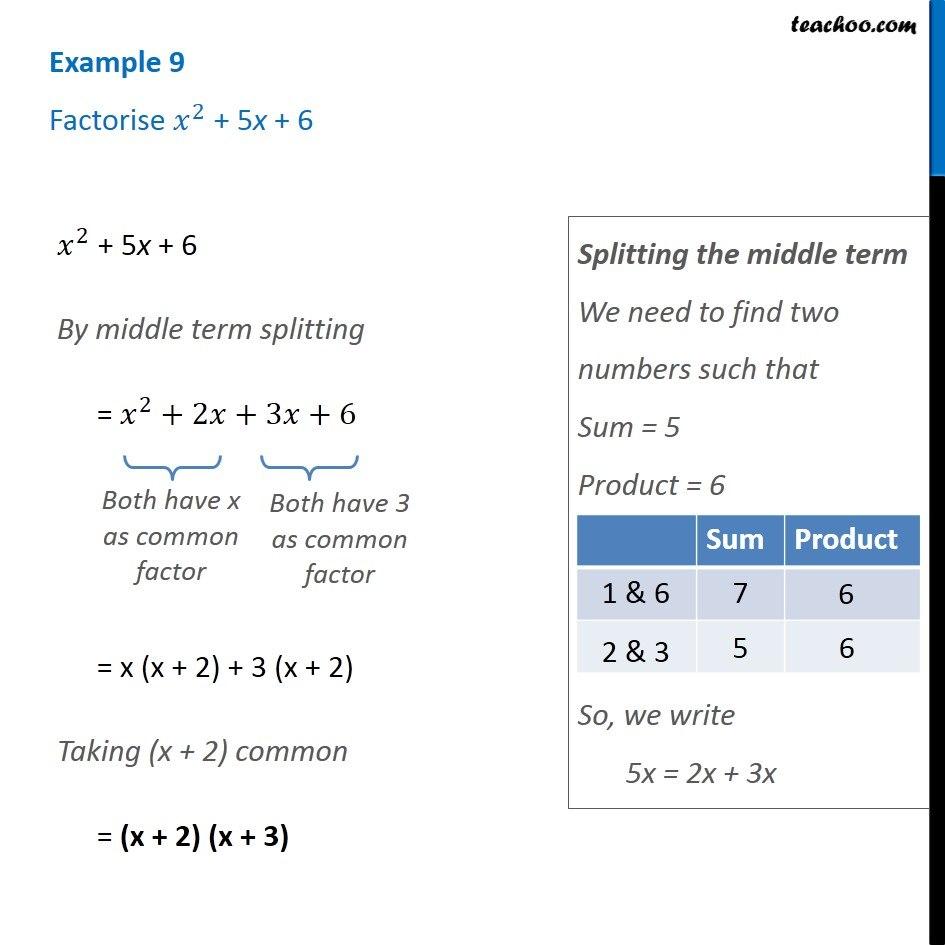Factorisation using splitting middle term

Chapter 14 Class 8 Factorisation
Concept wiseIntroducing your new favourite teacher - Teachoo Black, at only ₹83 per month

### Transcript

Example 9 Factorise 𝑥^2 + 5x + 6 𝑥^2 + 5x + 6 By middle term splitting = 𝑥^2+2𝑥+3𝑥+6 Both have x as common factor Both have 3 as common factor = x (x + 2) + 3 (x + 2) Taking (x + 2) common = (x + 2) (x + 3) Splitting the middle term We need to find two numbers such that Sum = 5 Product = 6 So, we write 5x = 2x + 3x